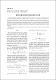## Періодичні біотопні простори2018
Вознюк, Оксана
Олійник, Богдана
Яворський, Роман
##### Abstract
У статтi введено узагальнення бiотопної метрики на нескiнченний випадок. Побудовано родину перiодичних бiотопних просторiв, елементами яких є перiодичнi {0, 1}-послiдовностi, перiоди яких є дiльниками супернатуральних чисел. Причому введена метрика мiж двома такими перiодичними послiдовностями не залежить вiд вибору спiльного перiоду. Доведено, що перiодичнi бiотопнi простори природним чином параметризуються супернатуральними числами. Точнiше, родина цих просторiв утворює вiдносно операцiї включення решiтку, iзоморфну решiтцi супернатуральних чисел. Кожен iз введених таким чином просторiв є iнварiантним вiдносно зсуву, тобто зсув є iзометрiєю для довiльного з таких просторiв.
Biotope spaces were introduced by Marchevsky-Steinhaus in  for the needs of mathematical biology, namely the study of ecosystems. Biotope distance is defined on the set of all subsets of some finite set X. The distance between any subsets A1 and A2 of X is calculated by the rule: d(A1, A2) = ( 0, if A1 = A2 = ∅; |A1⊕A2| |A1∪A2| , if A1, A2 ∈ B(X). We introduce a new generalization of a biotope metric to the infinite case using supernatural or Steinitz numbers. A supernatural number (or Steinitz number) is an infinite formal product of the form Y p∈P p kp where P is the set of all primes and kp ∈ N ∪ {0, ∞}. On the set of all periodic {0, 1}-sequences with the period that is a divisor of some supernatural u; we define the metric dB for any infinite periodic sequences x¯ and y¯ by the rule: dB(¯x, y¯) = dBn (¯xn, y¯n) where n is a common period of periodic sequences x¯ and y¯, and the formula dB(¯xn, y¯n) denotes the biotope distance between the first n coordinates of sequences x¯ and y¯ in the finite biotope metric space Bn. We denote the periodic biotope space that is defined by some Steinitz number u as B(u). If u is a finite Steinitz number, i.e. u is a positive integer, then B(u) is isometric finite biotope space Bu. We also prove that the introduced metric between such two periodic sequences does not depend on a choice of a common period. A family of such introduced periodic biotope spaces is naturally parametrized by supernatural numbers. More precisely, the family of these spaces forms a lattice that is isomorphic to the lattice of supernatural numbers. Moreover, each of these spaces B(u) is invariant with respect to the shift. We prove that the diametr of any periodic biotope space equals 1. We also show that any finite subset of a countable biotope space introduced in  is isometric embedding in the periodic biotope space B(u) for any u.
##### Keywords
метричний простiр, бiотопний простiр, супернатуральнi числа, перiодична послiдовнiсть, iзометрiя, стаття, metric spase, Biotope space, supernatural number, periodic sequence, isometry
##### Citation
Вознюк О. М. Періодичні біотопні простори / Вознюк О. М., Олійник Б. В., Яворський Р. С. // Могилянський математичний журнал : науковий журнал. - 2018. - Т. 1. - С. 6-10.